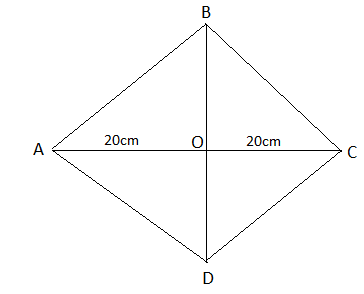QuestionAnswers

# The perimeter of a rhombus is 100 cm and one of the diagonal is 40 cm. Then the area of the rhombus is:A. $1000c{{m}^{2}}$B. $500c{{m}^{2}}$C. $1200c{{m}^{2}}$D. $600c{{m}^{2}}$Hint: We know that all sides of a rhombus are equal to each other. So we can get a side by dividing the perimeter by 4. Then we will use Pythagoras theorem to find the second diagonal of the rhombus and we will use the formula of area of rhombus which is equal to $\dfrac{1}{2}$ (products of diagonals).

We have been given the perimeter of the rhombus is 100 cm and one of the diagonals is 40 cm.
Let us suppose a rhombus ABCD having AC=40cm and their diagonal cuts at O.Since all the sides of a rhombus are equal to each other so we can get the side of a rhombus by dividing the perimeter by 4.
$\Rightarrow$Side of perimeter $=\dfrac{100}{4}cm=25cm$
Now in $\Delta ABO$, we have
AB = 25cm
Since it is a side of a rhombus,
AO = 20cm
Since it is half the diagonal
Now, we know that in a right angle triangle, the square of hypotenuse is equal to the sum of the square of perpendicular and base of the triangle, known as Pythagoras theorem.
So in $\Delta ABO$, we have
\begin{align} & A{{B}^{2}}=O{{B}^{2}}+O{{A}^{2}} \\ & {{\left( 25 \right)}^{2}}=O{{B}^{2}}+{{\left( 20 \right)}^{2}} \\ & 625=O{{B}^{2}}+400 \\ & 625-400=O{{B}^{2}} \\ & 225=O{{B}^{2}} \\ \end{align}
Taking square root on both the sides, we get as follows:
\begin{align} & \sqrt{225}=\sqrt{O{{B}^{2}}} \\ & 15cm=OB \\ \end{align}
Hence the second diagonal $=\left( 2\times 15 \right)cm=30cm$
Now we have the diagonals of rhombus are 40 cm and 30 cm.
We know that area of a rhombus having diagonals ${{d}_{1}}$ and ${{d}_{2}}$ is given as follows:
Area $=\dfrac{1}{2}\left( {{d}_{1}}\times {{d}_{2}} \right)$
So, area $=\dfrac{1}{2}\times 40\times 30c{{m}^{2}}=\dfrac{1}{2}\times 1200c{{m}^{2}}=600c{{m}^{2}}$
Therefore, the correct option of the above question is option D.

Note: Sometimes we just substitute the value of OB = 15cm in place of the value of the second diagonal during the calculation of area of the rhombus but the diagonal is twice of the OB. So be careful at this point. Also, remember that the perimeter of any geometrical 2-D figures is equal to the sum of the length of its sides.
View Notes
Area of a Rhombus FormulaThe Difference Between an Animal that is A Regulator and One that is A ConformerThe Perimeter of Rectangle FormulaPerimeter of a Trapezoid FormulaTable of 100 - Multiplication Table of 100What is the full form of phd?The Making of a ScientistChanging the Period of a PendulumCBSE Class 7 Maths Chapter 7 - Congruence of Triangles FormulasTable of 7 - Multiplication Table of 7Important Questions for CBSE Class 7 Maths Chapter 11 - Perimeter and AreaImportant Questions for CBSE Class 7 English Honeycomb Chapter 7 - The Invention of Vita - WonkImportant Questions for CBSE Class 7 English Honeycomb Chapter 10 - The Story of CricketImportant Questions for CBSE Class 6 English A Pact with The Sun Chapter 7 - The Wonder Called SheepImportant Questions for CBSE Class 11 Business Studies Chapter 7 - Formation of a CompanyImportant Questions for CBSE Class 6 English A Pact with The Sun Chapter 1 - A Tale of Two BirdsImportant Questions for CBSE Class 6 English A Pact with The Sun Chapter 6 - The Monkey and the CrocodileCBSE Class 8 Science Reaching The Age of Adolescence WorksheetsImportant Questions for CBSE Class 7 English Honeycomb Chapter 2 - A Gift Of ChappalsImportant Questions for CBSE Class 7 Social Science - Social and Political Life Chapter 8 - A Shirt In The MarketPrevious Year Question Paper of CBSE Class 10 EnglishCBSE Class 10 Hindi A Question Paper 2020Hindi A Class 10 CBSE Question Paper 2009Hindi A Class 10 CBSE Question Paper 2015Hindi A Class 10 CBSE Question Paper 2007Hindi A Class 10 CBSE Question Paper 2013Hindi A Class 10 CBSE Question Paper 2016Hindi A Class 10 CBSE Question Paper 2012Hindi A Class 10 CBSE Question Paper 2010Hindi A Class 10 CBSE Question Paper 2008NCERT Solutions for Class 7 Maths Chapter 11 Perimeter and AreaRS Aggarwal Class 10 Solutions - Perimeter and Areas of Plane FiguresRD Sharma Class 12 Maths Solutions Chapter 7 - Adjoint and Inverse of a MatrixNCERT Solutions for Class 7 Maths Chapter 7Weather, Climate and Adaptations of Animals to Climate NCERT Solutions - Class 7 ScienceRS Aggarwal Class 12 Solutions Chapter-7 Adjoint and Inverse of a MatrixRD Sharma Class 7 Solutions Chapter 7 - Algebraic Expressions (Ex 7.4) Exercise 7.4RD Sharma Class 7 Solutions Chapter 7 - Algebraic Expressions (Ex 7.2) Exercise 7.2RS Aggarwal Solutions Class 7 Chapter-7 Linear Equations in One Variable (Ex 7A) Exercise 7.1RS Aggarwal Solutions Class 7 Chapter-7 Linear Equations in One Variable (Ex 7B) Exercise 7.2